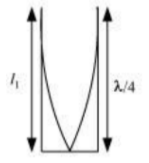15.15 A one metre-long tube open at one end, with a movable piston at the other end, shows resonance with a fixed frequency source (a tuning fork of frequency 340 Hz) when the tube length is 25.5 cm or 79.3 cm. Estimate the speed of sound in air at the temperature of the experiment. The edge effects may be neglected.

Frequency of the turning fork,
The pipe will behave as a pipe with one end closed and the other end open, as shown in the given figure.The pipe produces odd harmonics. The fundamental note in a closed pipe is given by:

${\mathrm{l}}_{\mathrm{l}}=\frac{\mathrm{\lambda }}{4}$

$\mathrm{where},$